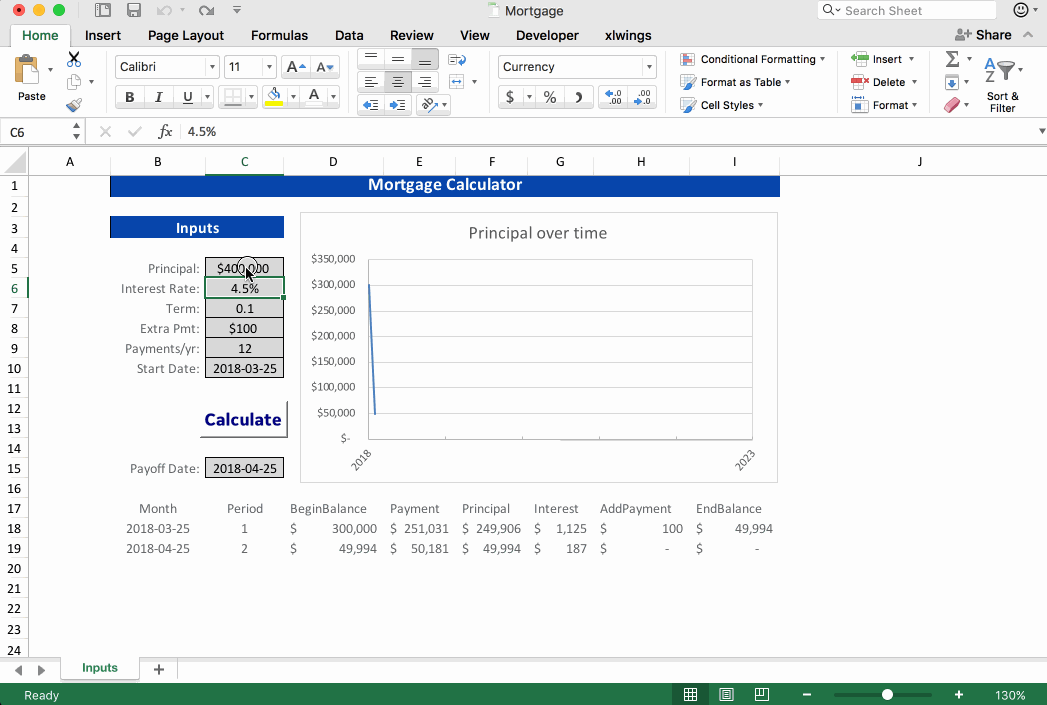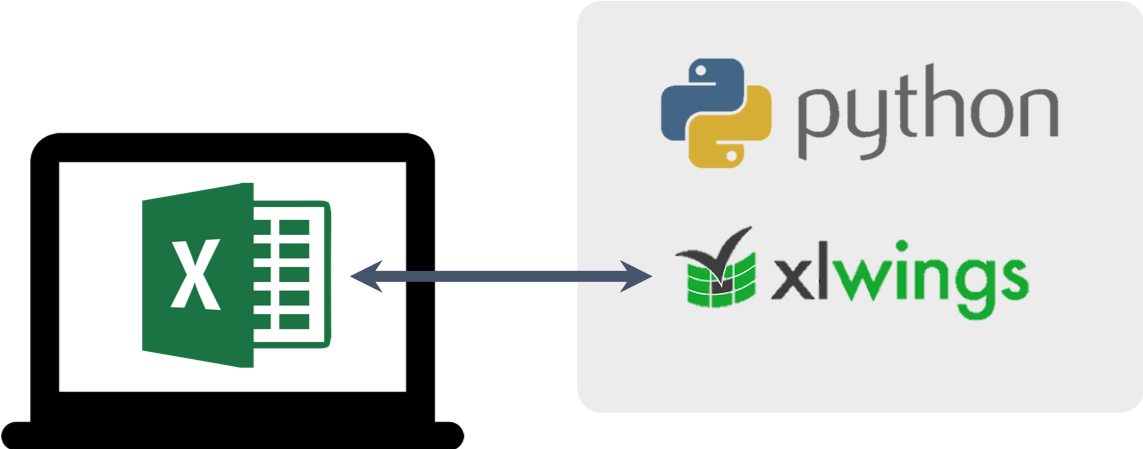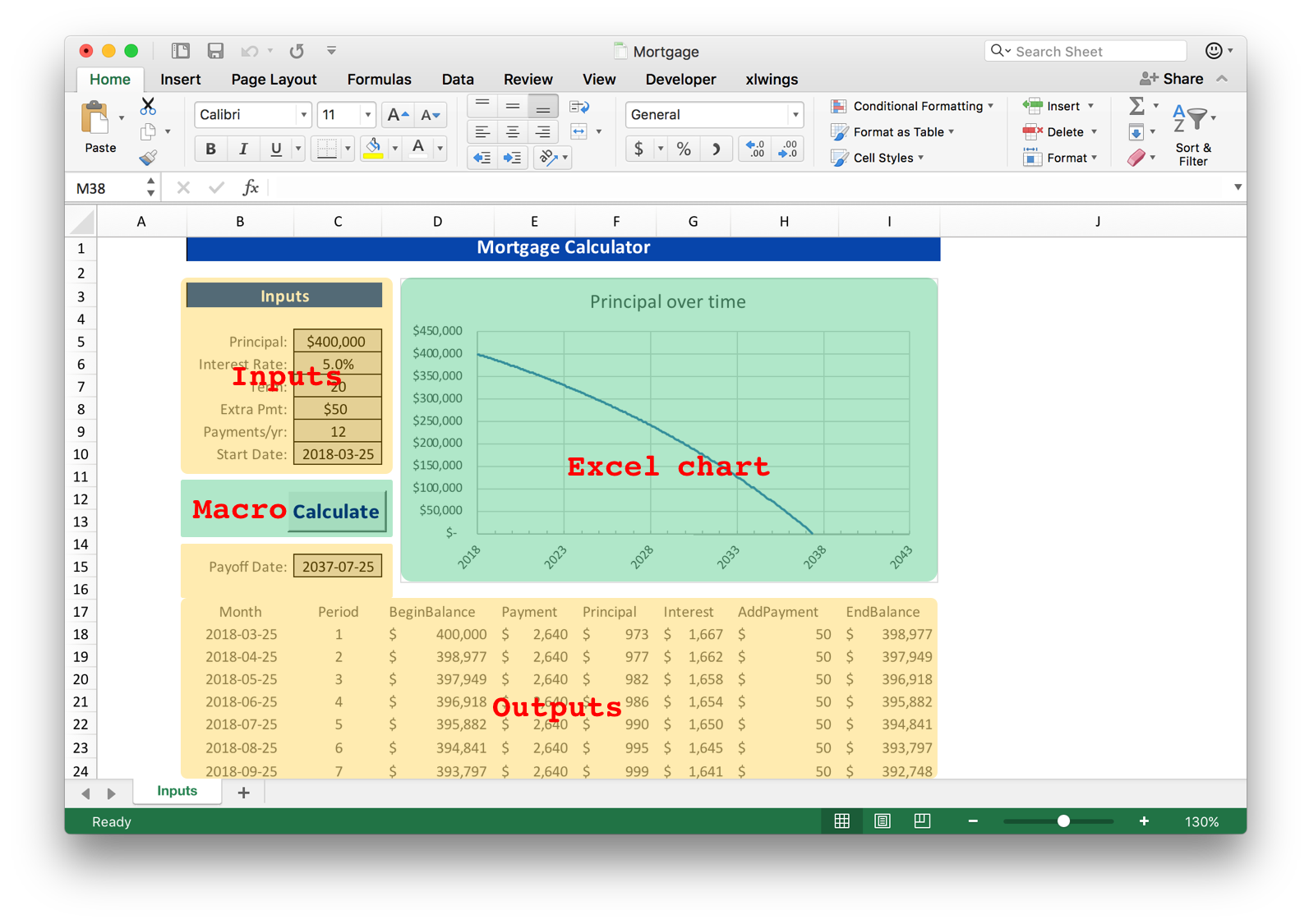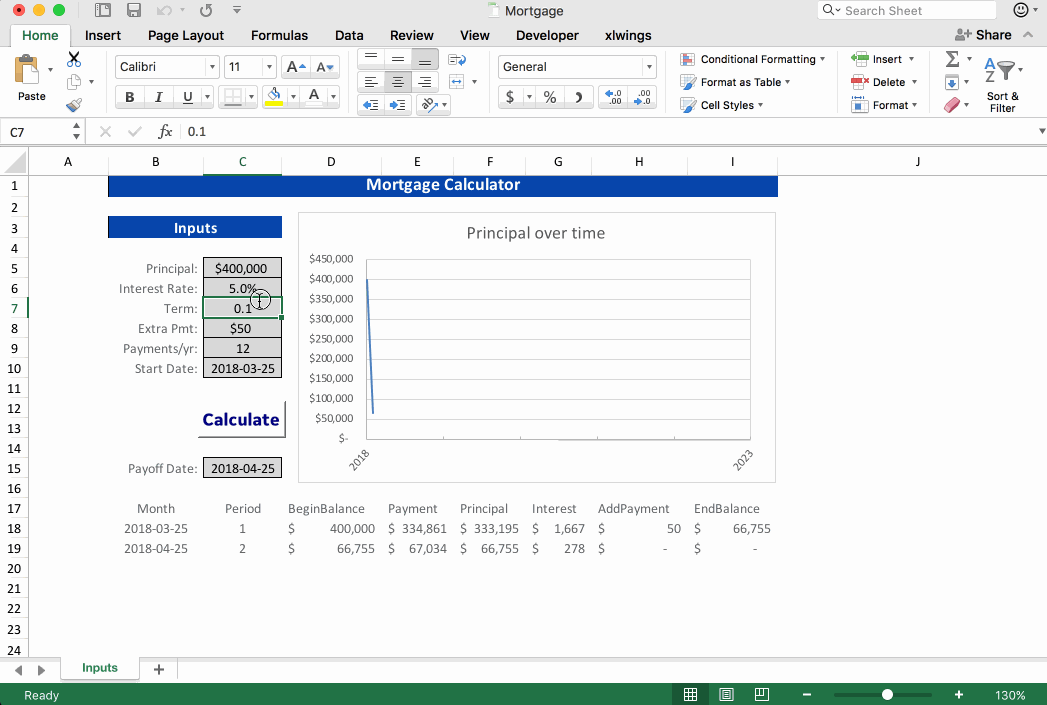# xlwings: Use Python and Excel to Calculate Your MortgageThe Python xlwings library provides easy interaction between Python and a Microsoft Excel workbook. In this example, I'll demonstrate using Excel as a wrapper around a Python function, with xlwings providing the link between the two.

# When to use xlwings

Python and Excel have complementary strengths and it can be useful to use them together. Excel is great for lightweight data exploration where you need some interactivity. Since Excel is the default tool for data analysis in the corporate world an average user is probably much less intimidated by an Excel spreadsheet than a .py or .ipynb file. Python provides heavy-duty analytics, has thousands of libraries freely available to add functionality, and allows you to make your analysis more reproducable since it can be version-controlled and quickly re-run with new data.

Great reasons to use xlwings include:

• Extending Excel: Let's face it—Excel has limits. When you want to run a loop or add some additional analytics power to Excel without writing VBA, Python is a great option.
• Python Front End: For all of Python's power there are situations where it can be tedious to continually interact with a function. While there are much more elegant (and Python-native) solutions available, Excel can be a low-overhead front end for interacting with Python in certain situations.
• Sharing: We already acknowledged that most people are more comfortable with Excel than Python. But in my experience, average Excel users are perfectly alright to use a tool in Excel that uses some Python behind the scenes (and even intrigued by the experience), as long as the proper support is provided to get them up and running.

Using xlwings to link Excel and Python can bring the best of both worlds. Let's get started.

# Wrapping a Python Function

The easiest way to get started with xlwings is to download and modify a working example. There are great examples on the xlwings page, or you can download all the files used to create this example here.

## Basic Structure

When all set up, our project directory will have two files:

├── Mortgage.xlsm
└── mortgage.py


Notice that Mortgage.xlsm is a macro-enabled Excel workbook. A macro—assigned to a button—will be used to initiate the the Python script. Our Python file mortgage.py contains our function, as well as the xlwings code to read from and write to the Excel workbook.## Python Function

The Python function we will use as an example is a financial model in pandas written by Chris Moffitt. We will take this function as an input, but please see Chris' original post for the details. This model builds a loan amortization table, such as you would need to evaluate a mortgage. Given the required inputs of loan principal, interest rate, term, etc. this Python function returns a pandas DataFrame giving the amortization table.

import pandas as pd
from datetime import date
import numpy as np
from collections import OrderedDict
from dateutil.relativedelta import *

def amortize(principal, interest_rate, years, addl_principal=0, annual_payments=12, start_date=date.today()):
"""
The below code, which may have been edited, was originally written by
Chris Moffitt of pbpython.com (used with permission):
- Blog Post: http://pbpython.com/amortization-model-revised.html
- Code: https://github.com/chris1610/pbpython/blob/63e810a42d30c8297b82f6da43e5e962b8a6f15a/notebooks/Amortization-Corrected-Final.ipynb
"""

pmt = -round(np.pmt(interest_rate/annual_payments, years*annual_payments, principal), 2)
# initialize the variables to keep track of the periods and running balances
p = 1
beg_balance = principal
end_balance = principal

while end_balance > 0:

# Recalculate the interest based on the current balance
interest = round(((interest_rate/annual_payments) * beg_balance), 2)

# Determine payment based on whether or not this period will pay off the loan
pmt = min(pmt, beg_balance + interest)
principal = pmt - interest

# Ensure additional payment gets adjusted if the loan is being paid off
end_balance = beg_balance - (principal + addl_principal)

yield OrderedDict([('Month',start_date),
('Period', p),
('BeginBalance', beg_balance),
('Payment', pmt),
('Principal', principal),
('Interest', interest),
('EndBalance', end_balance)])

# Increment the counter, balance and date
p += 1
start_date += relativedelta(months=1)
beg_balance = end_balance


Remember that while we are using a financial model as an example, just about anything you can do in Python is fair game for extending the capabilities of Excel.

## Set up the workbook

First, let's set up the Excel workbook. Our user will set values for the required inputs, press the "Calculate" button, and see outputs written by xlwings. The chart is a native Excel chart constructed off the table in the outputs, but xlwings can write image files to Excel so you could generate charts in matplotlib or another Python plotting library as well. This is a standard .xlsm macro-enabled Excel workbook, so you can apply formatting and add charts as you like.## Set up Python with xlwings

Our Python script mortgage.py uses xlwings to interface with Excel. First we include the amortize() function discussed previously. All the xlwings for interacting with the workbook is in the main() function. Here we define the sheet(s) to pull from, clear previous values as necessary, perform calculations, and write calculated values to Excel.

def amortize(principal, interest_rate, years, addl_principal=0, annual_payments=12, start_date=date.today()):
"""
Function shown above
"""

# --------------------------------------------------------------------------------------------------------
import xlwings as xw
import datetime as dt

# --------------------------------------------------------------------------------------------------------
def main():
# define sheet(s)
sht = xw.Book.caller().sheets['Inputs']

# clear sheet(s)
sht.range('A17').expand().clear_contents()

principal       = sht.range('C5').value
interest_rate   = sht.range('C6').value
years           = sht.range('C7').value
annual_payments = sht.range('C9').value
start_date      = sht.range('C10').options(dates=dt.date).value

# perform calcs
schedule = pd.DataFrame(amortize(principal, interest_rate, years, addl_principal, annual_payments, start_date))
payoff_date = schedule['Month'].max()

# write values to Excel
sht.range('C15').value = payoff_date
sht.range('A17').value = schedule

if __name__ == '__main__':
# Expects the Excel file next to this source file, adjust accordingly.
xw.Book('Mortgage.xlsm').set_mock_caller()
main()


## Set up the macro

Finally, map the the Calculate button to the Button_click() macro to call mortgage.py.

Sub Button_click()
RunPython ("import mortgage; mortgage.main()")
End Sub


Note that in this macro mortgage is the name of our script, and main is the function to call from within our script. You can easily have different buttons call different functions from the same script. This can be helpful for more complicated workbooks. For example, with multiple buttons you could load data with one, enter values or modify the data, and then use another button to load the modified data and perform final calculations.

## Run it

Running the workbook is as simple as updating your inputs and clicking Calculate.You will notice the if __name__ == '__main__': statement at the end of mortgage.py. This allows us to run the script by calling python mortgage.py from the command line instead of pressing the Calculate button in the workbook. This can be especially helpful for debugging when you want to print values or read error messages.# Closing Thoughts

I hope this illustrates the ability to connect Excel to Pyton and prompts you to think about useful applications for this. All that is needed is a bit of glue to hold the two together, for which xlwings is a great tool. To learn more about xlwings and other options for interacting with worksheets using Python, have a look at the links below:

© 2005 Matthew Kudija | Source